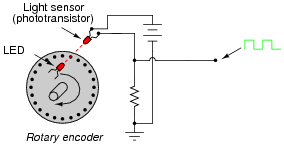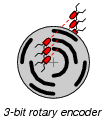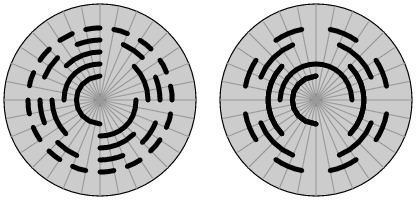# Digital Codes

## Digital Circuits

• #### Question 1

Counting practice: count from zero to thirty-one in binary, octal, and hexadecimal:• #### Question 2

Rotary encoders are electromechanical devices used to convert an angular position (shaft rotation) into a digital signal. The simplest form of rotary encoder uses a slotted wheel with a single LED/photodetector pair to generate pulses as the wheel turns:Some rotary encoder designs have multiple-bit outputs, with each LED/photodetector pair reading a different “track” of slots in the disk:In the illustration shown above, identify which LED/photodetector pairs represent the MSB (Most Significant Bit) and LSB (Least Significant Bit) of the binary output. Also, identify which direction the wheel must turn in order to produce an increasing count.

Note: assume that the darkest areas on the illustration represent slots cut through the disk, while the grey areas represent parts of the disk that are opaque.

• #### Question 3

Absolute rotary encoders often use a code known as Gray code rather than binary, to represent angular position. This code was patented by Frank Gray of Bell Labs in 1953, as a means of reducing errors in rotary encoder output. Examine each of these encoder disks, and determine which one is binary and which one is Gray code:Assuming that the darkest areas on the illustration represent slots cut through the disk, and the grey areas represent parts of the disk that are opaque, mark the “zero,” “one,” and “two” sectors on each disk.

• #### Question 4

Explain why Gray code is often used in rotary encoders rather than binary coding. What difference does it make what type of code we use to mark the sectors of an encoder disk, so long as each sector possesses a unique number?

• #### Question 5

Convert the following binary numbers into Gray code:

1001102 =
1100102 =
1010012 =
10101001102 =
• #### Question 6

Convert the following Gray code numbers into binary:

111110Gray =
100001Gray =
101110Gray =
1110001111Gray =
• #### Question 7

A nearly universal standard for representing text data in digital form is the ASCII code. What does the acronym “ASCII” stand for, and what is the format of this code?

• #### Question 8

Decode this set of ASCII characters, to reveal a secret message (all codes given in hexadecimal format):

49 20 4C 6F 76 65 20 45 6C 65 63 74 72 6F 6E 69 63 73 21

• #### Question 9

Explain why Binary Coded Decimal (BCD) is sometimes referred to as the “8421” code. Why is this code used at all?

• #### Question 10

Convert the following decimal numbers into BCD form:

73910
2510
9224110

Convert the following BCD numbers into decimal form:

1000 1001
0100 0111 0110
0011 1000 0101 0001